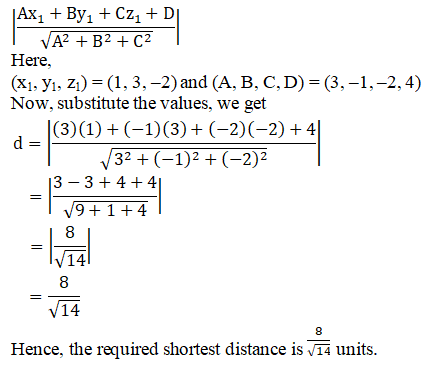# RD Sharma Solutions Class 12 The Plane Exercise 29.14

RD Sharma Solutions for Class 12 Maths Chapter 29 The Plane Exercise 29.14, is provided here. The solutions are mainly designed for students to grasp the concepts quickly and have a strong command over all the subjects. To know the correct methodology to answer any question, then quickly refer to RD Sharma Solutions. By referring to the answers provided by the subject-matter experts, students can excel in their examinations. Download the solutions pdf for free from the links given below.

## Download PDF of RD Sharma Solutions For Class 12 Maths Chapter 29 Exercise 14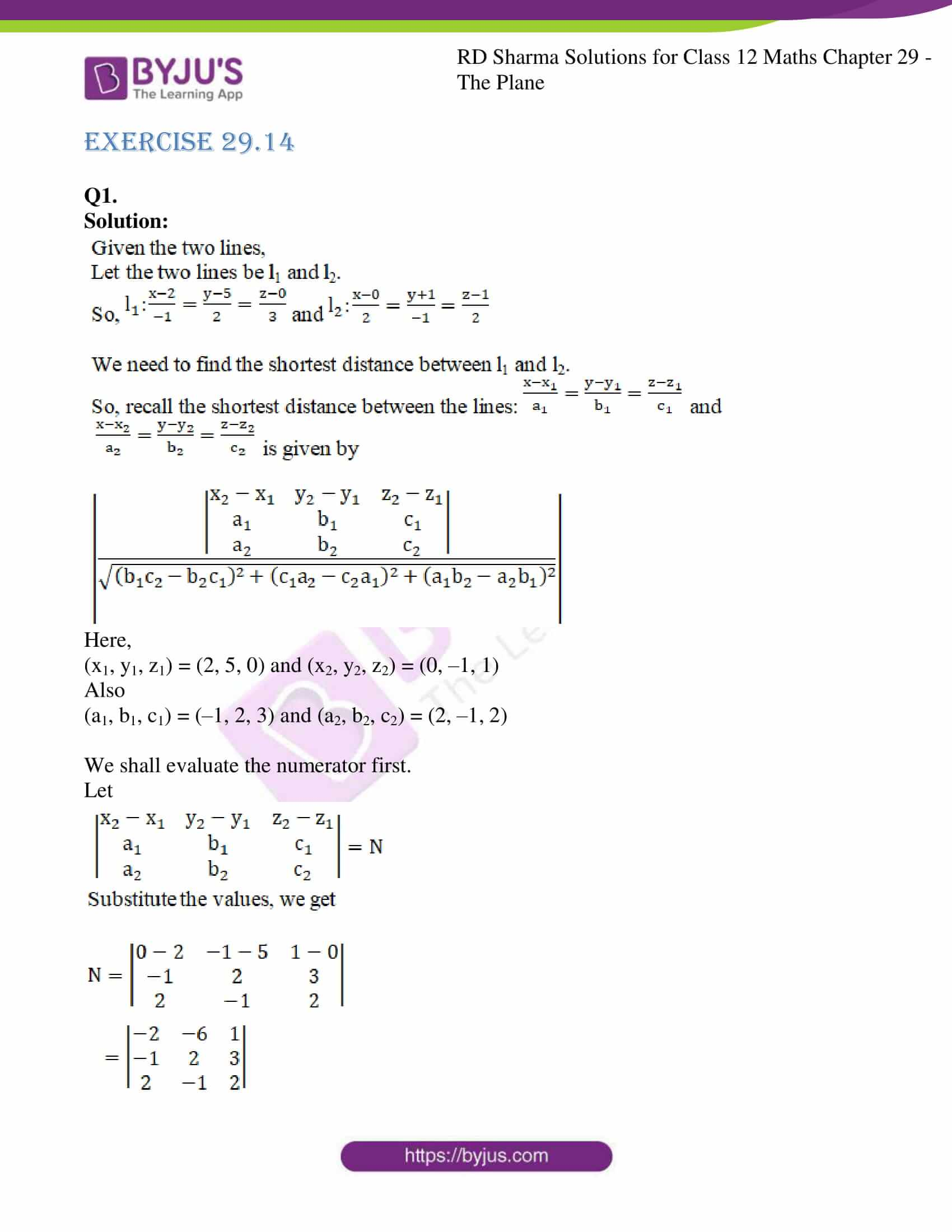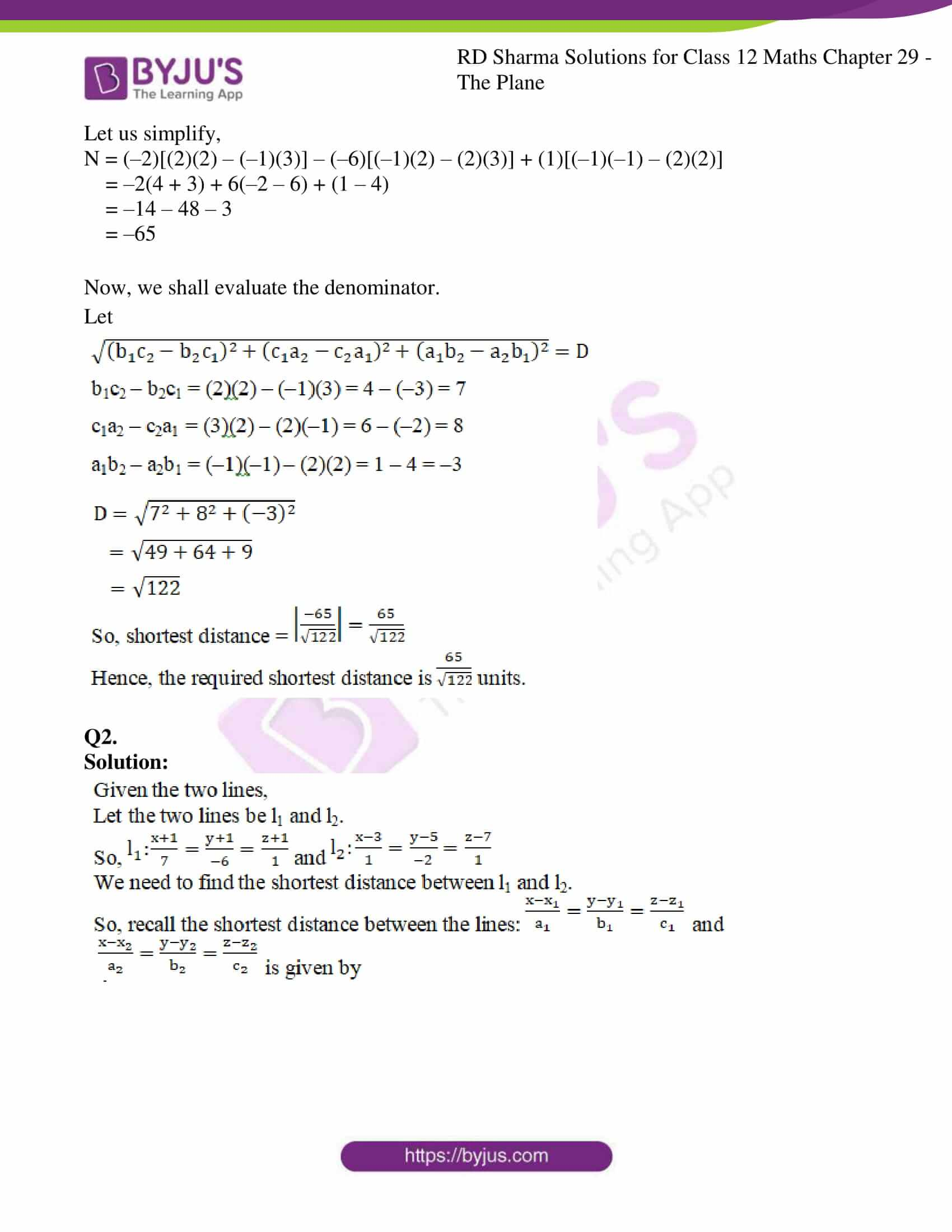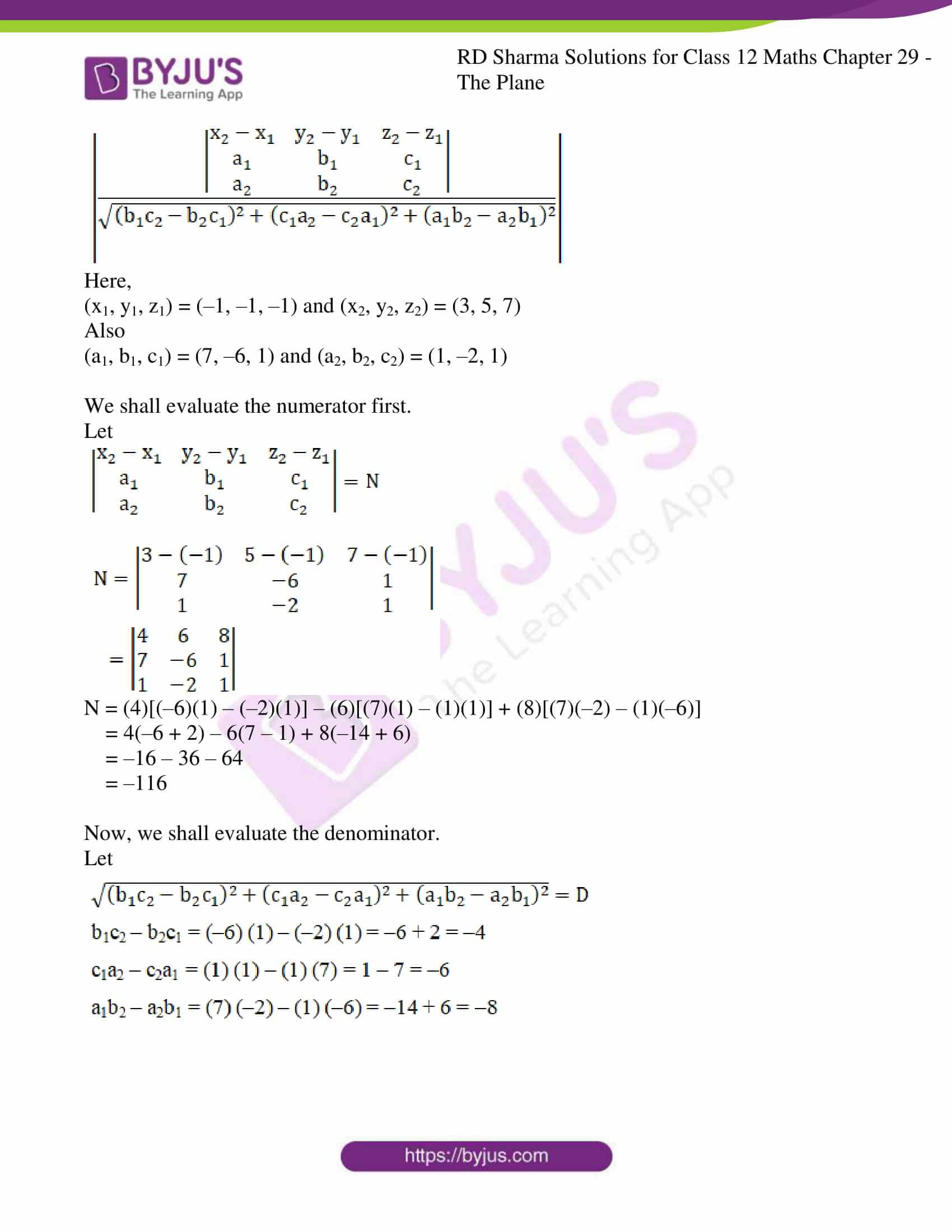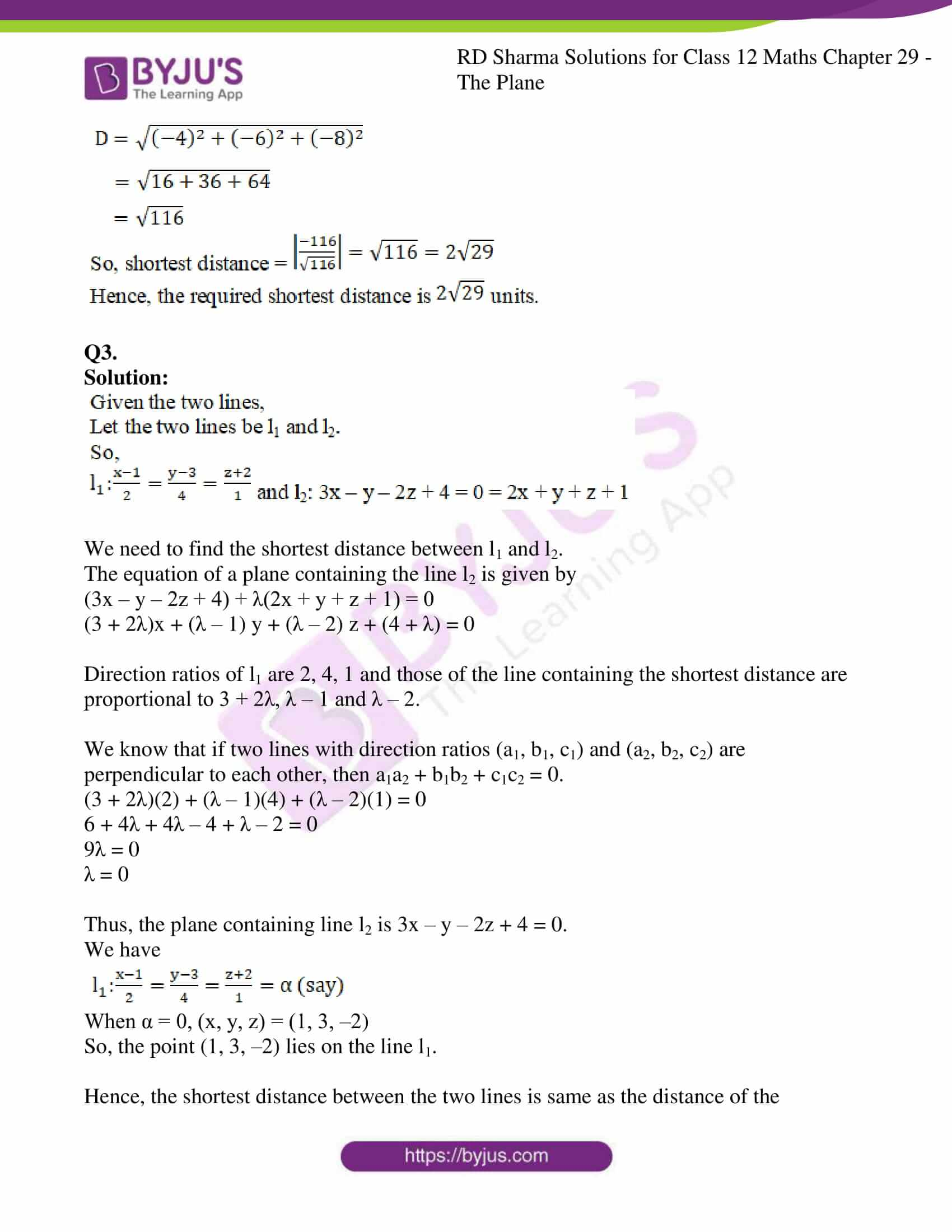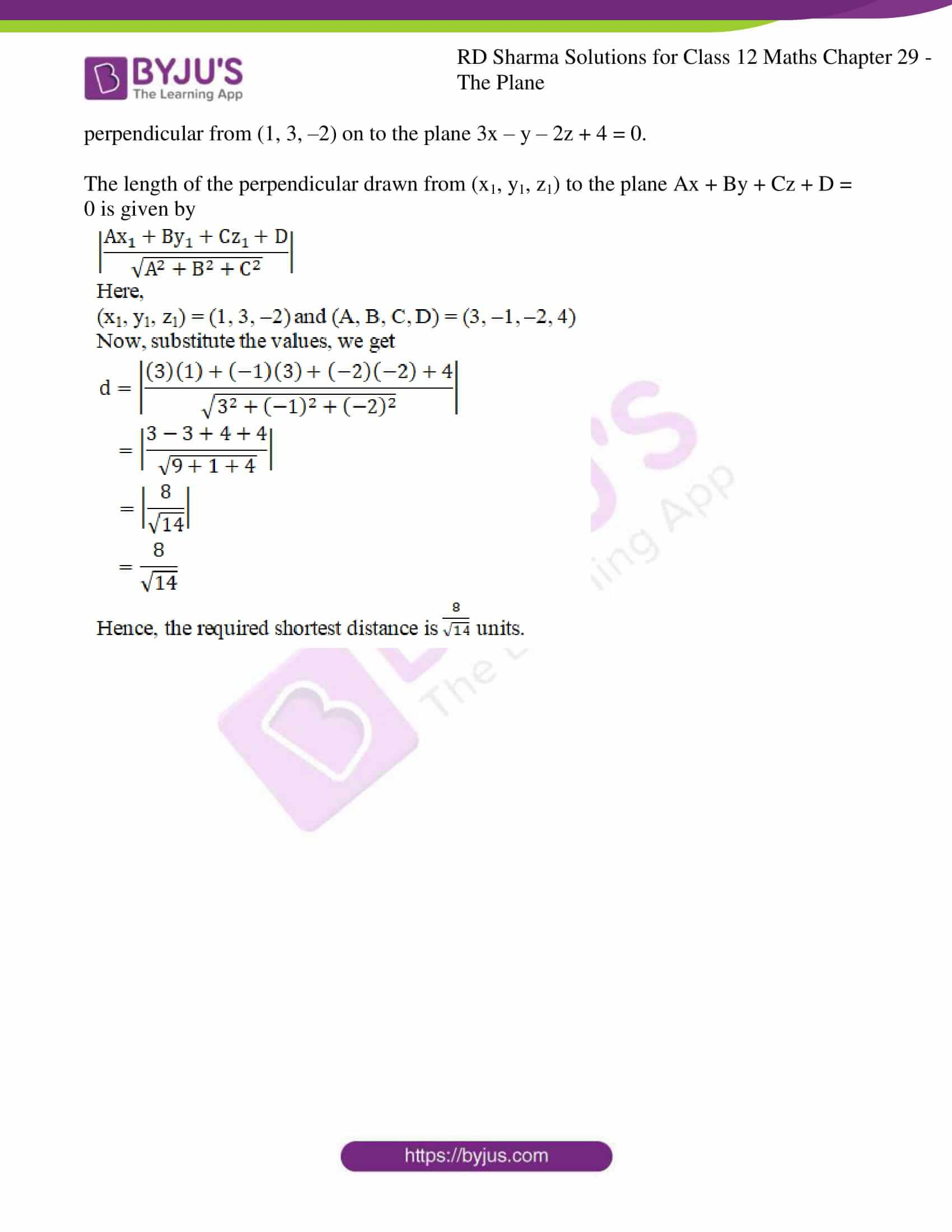### Access RD Sharma Solutions For Class 12 Maths Chapter 29 Exercise 14

EXERCISE 29.14

Q1.

Solution: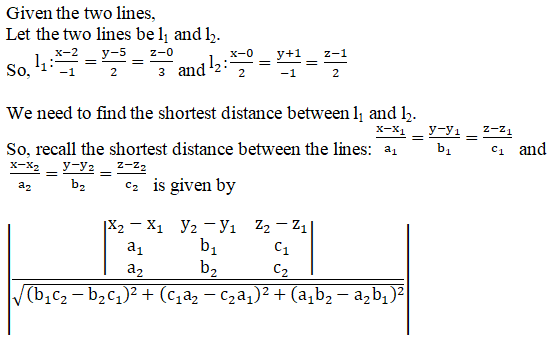Here,

(x1, y1, z1) = (2, 5, 0) and (x2, y2, z2) = (0, –1, 1)

Also

(a1, b1, c1) = (–1, 2, 3) and (a2, b2, c2) = (2, –1, 2)

We shall evaluate the numerator first.

Let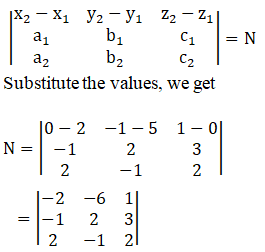Let us simplify,

N = (–2)[(2)(2) – (–1)(3)] – (–6)[(–1)(2) – (2)(3)] + (1)[(–1)(–1) – (2)(2)]

= –2(4 + 3) + 6(–2 – 6) + (1 – 4)

= –14 – 48 – 3

= –65

Now, we shall evaluate the denominator.

Let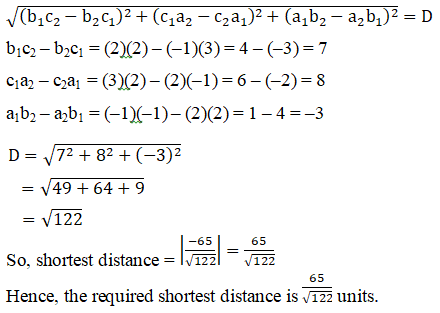Q2.

Solution: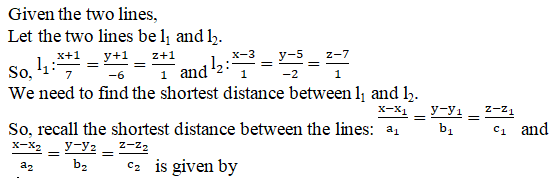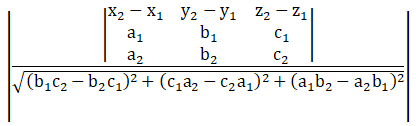Here,

(x1, y1, z1) = (–1, –1, –1) and (x2, y2, z2) = (3, 5, 7)

Also

(a1, b1, c1) = (7, –6, 1) and (a2, b2, c2) = (1, –2, 1)

We shall evaluate the numerator first.

Let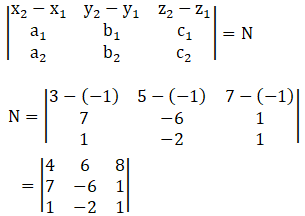N = (4)[(–6)(1) – (–2)(1)] – (6)[(7)(1) – (1)(1)] + (8)[(7)(–2) – (1)(–6)]

= 4(–6 + 2) – 6(7 – 1) + 8(–14 + 6)

= –16 – 36 – 64

= –116

Now, we shall evaluate the denominator.

Let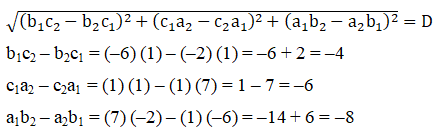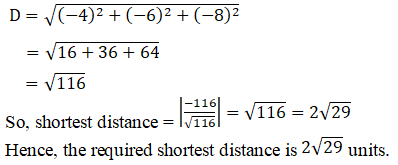Q3.

Solution: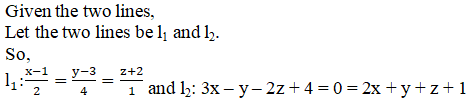We need to find the shortest distance between l1 and l2.

The equation of a plane containing the line l2 is given by

(3x – y – 2z + 4) + λ(2x + y + z + 1) = 0

(3 + 2λ)x + (λ – 1) y + (λ – 2) z + (4 + λ) = 0

Direction ratios of l1 are 2, 4, 1 and those of the line containing the shortest distance are proportional to 3 + 2λ, λ – 1 and λ – 2.

We know that if two lines with direction ratios (a1, b1, c1) and (a2, b2, c2) are perpendicular to each other, then a1a2 + b1b2 + c1c2 = 0.

(3 + 2λ)(2) + (λ – 1)(4) + (λ – 2)(1) = 0

6 + 4λ + 4λ – 4 + λ – 2 = 0

9λ = 0

λ = 0

Thus, the plane containing line l2 is 3x – y – 2z + 4 = 0.

We have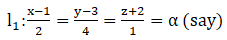When α = 0, (x, y, z) = (1, 3, –2)

So, the point (1, 3, –2) lies on the line l1.

Hence, the shortest distance between the two lines is same as the distance of the perpendicular from (1, 3, –2) on to the plane 3x – y – 2z + 4 = 0.

The length of the perpendicular drawn from (x1, y1, z1) to the plane Ax + By + Cz + D = 0 is given by